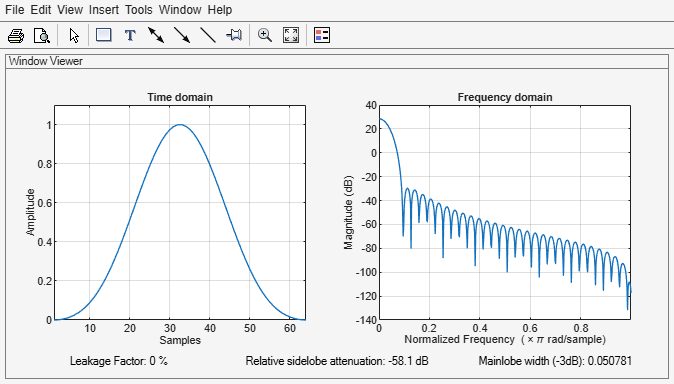# blackman

Blackman window

## Syntax

``w = blackman(L)``
``w = blackman(L,sflag)``

## Description

example

````w = blackman(L)` returns an `L`-point symmetric Blackman window.```
````w = blackman(L,sflag)` returns a Blackman window using the window sampling method specified by `sflag`.```

## Examples

collapse all

Create a 64-point Blackman window. Display the result using `wvtool`.

```L = 64; wvtool(blackman(L))```## Input Arguments

collapse all

Window length, specified as a positive integer.

Data Types: `single` | `double`

Window sampling method, specified as:

• `'symmetric'` — Use this option when using windows for filter design.

• `'periodic'` — This option is useful for spectral analysis because it enables a windowed signal to have the perfect periodic extension implicit in the discrete Fourier transform. When `'periodic'` is specified, the function computes a window of length `L` + 1 and returns the first `L` points.

## Output Arguments

collapse all

Blackman window, returned as a column vector.

## Algorithms

The following equation defines the Blackman window of length N:

`$w\left(n\right)=0.42-0.5\mathrm{cos}\left(\frac{2\pi n}{L-1}\right)+0.08\mathrm{cos}\left(\frac{4\pi n}{L-1}\right),\text{ }0\le n\le M-1$`

where M is N/2 when N is even and (N + 1)/2 when N is odd.

In the symmetric case, the second half of the Blackman window, M ≤ n ≤ N – 1, is obtained by reflecting the first half around the midpoint. The symmetric option is the preferred method when using a Blackman window in FIR filter design.

The periodic Blackman window is constructed by extending the desired window length by one sample to N + 1, constructing a symmetric window, and removing the last sample. The periodic version is the preferred method when using a Blackman window in spectral analysis because the discrete Fourier transform assumes periodic extension of the input vector.

 Oppenheim, Alan V., Ronald W. Schafer, and John R. Buck. Discrete-Time Signal Processing. Upper Saddle River, NJ: Prentice Hall, 1999, pp. 468–471.